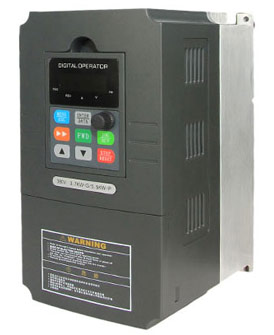Categories

# Can capacitors in VFD contribute to improve power factor?

Capacitors inside the variable frequency drive (VFD) is mainly used to maintain the DC voltage, this is common thing all are well known about this, but power factor is mainly improved due to the cosine angle between voltage and current are mostly near to each other, however the inductive load produces the reactive power which will directly affect the wave form of input of VFD sine wave when we used additional filter capacitors in VFD which will give more stability in DC wave form but output is not continuous sine wave. Here the output wave form of VFD is almost square wave more ever it is equal to sine wave when no of switching is more, when the power factor is more ever unity, means the output waveform of voltage or current must equal to input sine wave form. It is not possible in practical variable frequency drive.We can get rid of the AC ripple on the DC bus by adding a capacitor. A capacitor operates in a similar fashion to a reservoir or accumulator in a plumbing system. This capacitor absorbs the ac ripple and delivers a smooth dc voltage. The AC ripple on the DC bus is typically less than 3 Volts. Thus, the voltage on the DC bus becomes "approximately" 650VDC. The actual voltage will depend on the voltage level of the AC line feeding the drive, the level of voltage unbalance on the power system, the motor load, the impedance of the power system, and any reactors or harmonic filters on the VFD.

Power factor is mainly depends on in VFD output waveform however capacitors adding is not giving exact sine wave in output as equal to input, that means power factor is not depend on filter capacitors.

A VFD could always soft start a motor reducing the need for dynamic compensation, also since the VFD maintains the v/f ratio in proportion with the voltage core saturation and squared losses are reduced, so we do not need a capacitor in most cases, however there are high inertia loads which require full voltage start, in synch applications the capacitors are used only as dynamic compensators during the inrush and become isolated once the VFD is engaged.

VFDs , AC Drives